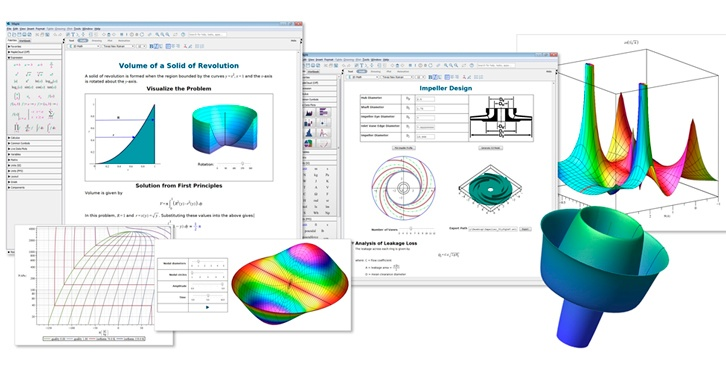## The Calculating Engineer

Presented by: Samir Khan, Product Manager, Maplesoft
Yu Shen, Senior Manager, Technical Analysis, Hitachi Contruction Truck Manufacturing

Calculations are the core of any engineered product. Calculations deserve respect and should be treated like any other asset. Well-implemented calculations are an investment – a little up-front care and attention means that you can reap the dividends of that work for years to come. Starting a calculation with a spreadsheet is easy - just simply fire up a new worksheet and start entering equations. But before you realise it, you’re face with hard-to-read equations and spaghetti logic that makes following the flow of a calculation difficult. You never get the most value out of the work you’ve done.

In this webinar we will discuss how modern tools can help engineers do, document and manage calculations in a way that maximizes return on investment. You will also discover how enterprising engineers at Hitachi Heavy Machinery in Guelph, Ontario are transforming decaying spreadsheets into easy-to-follow and maintainable calculation sheets.

Webinar Outline

• The fundamental characteristics of engineering calculations
• How spreadsheets encourage engineers to build unmaintainable, difficult to follow calculations
• How Maple helps engineers do and document calculations using natural math notation, text and graphics
• How enterprising engineers at Hitachi Heavy Machinery in Guelph, Ontario are transforming decaying spreadsheets into easy-to-follow and maintainable calculation sheets

Who Should Attend?

• Engineers, scientists and mathematicians involved in advanced analysis, applications development, validation and design calculations
• Managers of modeling and simulation projects, mathematical analysis of complex dataMaple makes it easy to analyze, explore, visualize, and solve mathematical problems